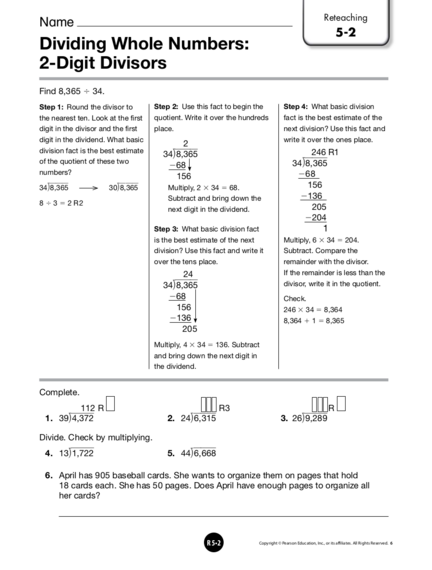# Dividing Whole Numbers: 2-Digit DivisorsContributed by:This is a complete lesson that includes examples and exercises about two-digit divisor in long division.
1. Reteaching
5-2
Dividing Whole Numbers:
2-Digit Divisors
Find 8,365 4 34.
Step 1: Round the divisor to Step 2: Use this fact to begin the Step 4: What basic division
the nearest ten. Look at the first quotient. Write it over the hundreds fact is the best estimate of the
digit in the divisor and the first place. next division? Use this fact and
digit in the dividend. What basic write it over the ones place.
2
division fact is the best estimate 246 R1
34​
8,365​
of the quotient of these two 34​
8,365​
268
numbers? 268
156
8,365​      30​
8,365​ Multiply, 2  34 5 68. 156
Subtract and bring down the 2136
8  3  2 R2
next digit in the dividend. 205
2204
Step 3: What basic division fact 1
is the best estimate of the next Multiply, 6  34 5 204.
division? Use this fact and write it Subtract. Compare the
over the tens place. remainder with the divisor.
24 If the remainder is less than the
34​
8,365​ divisor, write it in the quotient.
268 Check.
156 246 3 34 5 8,364
2136 8,364  1 5 8,365
205
Multiply, 4 3 34 5 136. Subtract
and bring down the next digit in
the dividend.
112 R R3   R
1. 39​
4,372​ 2. 24​
6,315​ 3. 26​
9,289​
Divide. Check by multiplying.
4. 13​
1,722​ 5. 44​
6,668​
6. April has 905 baseball cards. She wants to organize them on pages that hold
18 cards each. She has 50 pages. Does April have enough pages to organize all
her cards?